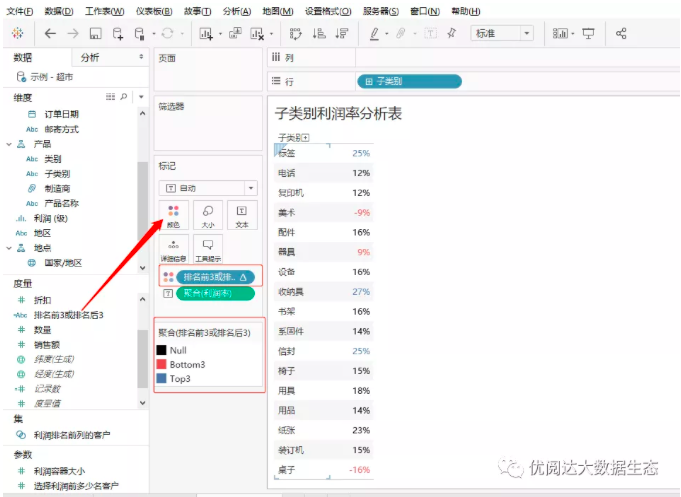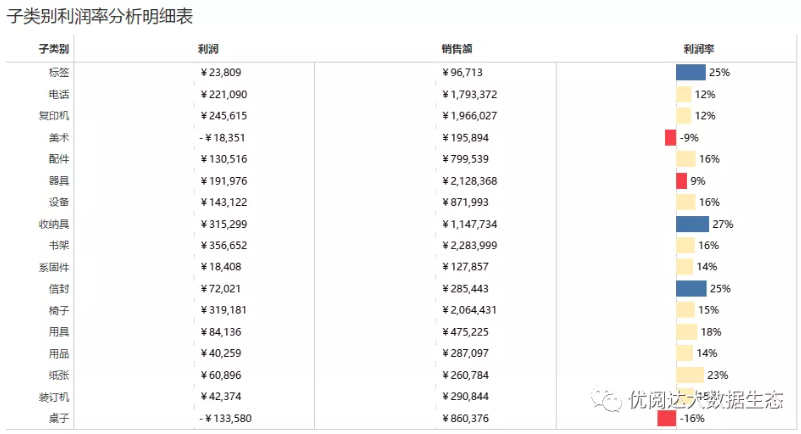published: 2021-09-17 16:56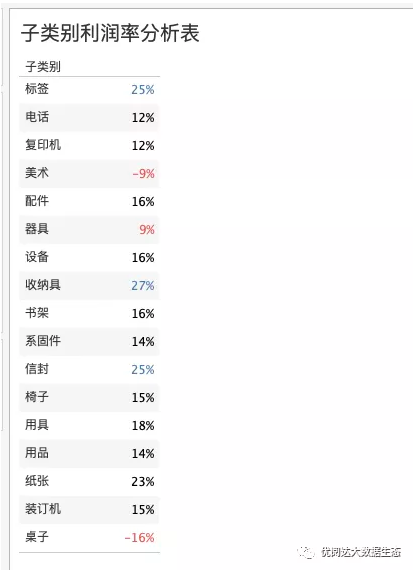Step.1

➤ 创建计算字段：利润率，键入函数

SUM([利润])/SUM([销售额])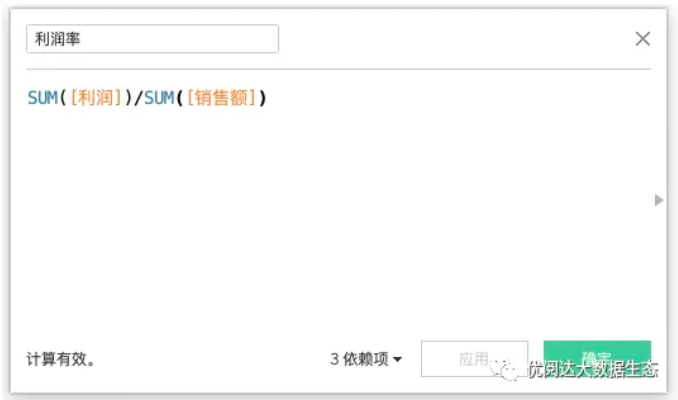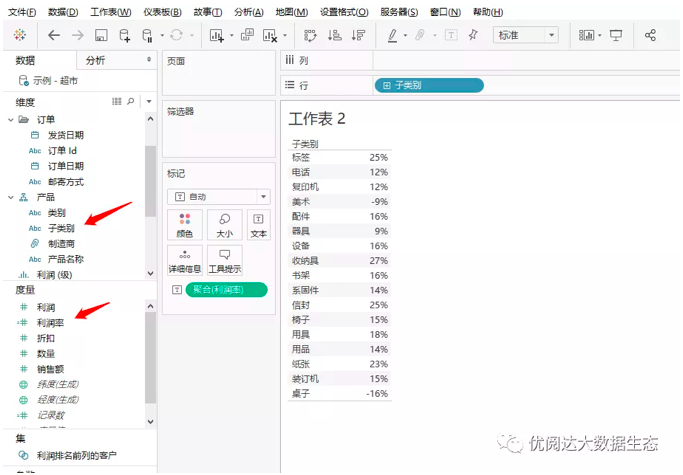Step.2

➤ 创建计算字段：排名前3或排名后3，键入函数

IF RANK_UNIQUE([利润率],'desc')=1

or

RANK_UNIQUE([利润率],'desc')=2

or

RANK_UNIQUE([利润率],'desc')=3

THEN "Top3"

ELSEIF RANK_UNIQUE([利润率],'asc')=1

or

RANK_UNIQUE([利润率],'asc')=2

or

RANK_UNIQUE([利润率],'asc')=3

THEN "Bottom3"

END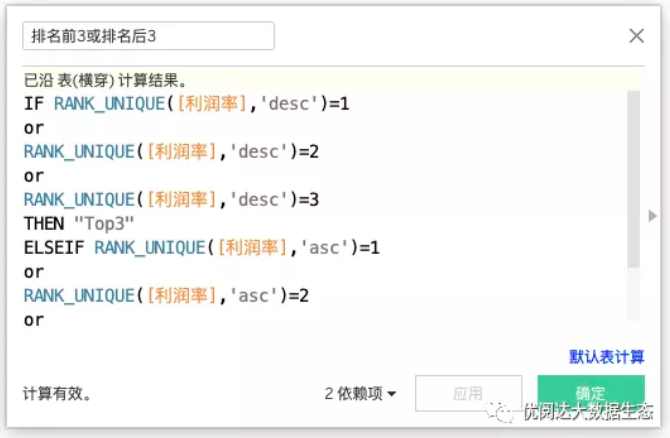Step.3